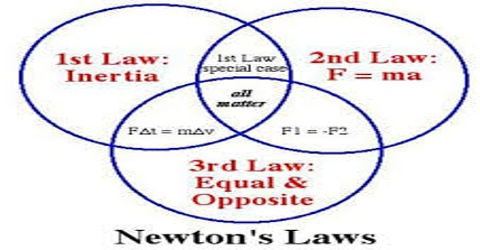# Relation between Newton’s Laws of Motion

Relation between Newton’s Laws of Motion

In order to establish relation between Newton’s laws of motion we should have enough idea about the laws and also we should have knowledge about the subjects on which those laws deal with.

First law: A body at rest will always tend to remain at rest and the body which is in motion will tend to remain in motion unless the state is being changed by external force.

Second law: Rate of change of momentum of a body is proportional to applied force on the body and the direction along which the force acts, the change of momentum also occurs along that direction.

Third law: Every action has equal and opposite reaction.

From the first law we understand that it no external influence acts on a body, the body will not change its state. Body at rest will continue to be at rest and the body in motion will continue to be in motion. This happens due to inertia of a body. In doing something against this inertia, i.e., to change a body at rest to move or to change the motion of a moving body, force is to be applied on the body.

From this idea, we can apply Newton’s second Law of motion. More the mass of a body, the more the momentum of that body. Suppose mass of a moving body is m and velocity is v; mass of another body is 2m but velocity is same, i.e., velocity is v.

Then momentum of the first body = mv and that of the second body is = 2 mv. By resisting i.e., by applying force if the two bodies are stopped at the same time then change of momentum of the second body will be double that of the hest body. From the second law, we know that rate of change of momentum a proportional to the applied force. So, in order to stop the second body at the same time double amount of force is to be applied.  Again, if two equal forces (F) are applied on the two bodies then acceleration of the first body is a1 and acceleration of the second body is a2, then according to Newton’s second law, F = ma1 and F = ma2.

So, it is seen that there is a relation between momentum and acceleration and by this a relation can be established between the first and the second law or one law can be transformed into the second law.

In other way, if forces F1 and F2 are applied on two bodies along the same straight line then while moving it may happen that the two bodies might have collided with each other. Whenever they are collided then second body apply equal and opposite reaction force on the first body. In this case, the force by which the second body is acted is called action force and this body after collision applies force on the first body in the opposite direction which is called reaction force. From Newton’s third law of motion it is known that this action and reaction are equal.